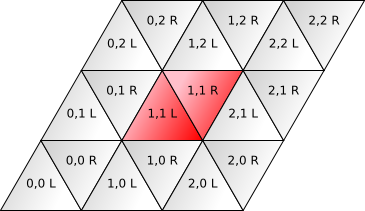As part of my article on grids, I'd like to provide basic algorithms for grids. I already know how to compute distances on square and hexagon grids, but I didn't know how to compute them on a triangle grid. I initially tried changing coordinate systems, inspired by Charles Fu's coordinate system. I normally use the coordinate system from my article on grids:The first coordinate (which I will call `u`) is the position along the horizontal axis; the second (which I call `v`) is the position along the southwest/northeast diagonal. The Left/Right parity introduces some compliation; I let `R` be 1 and `L` be 0. This coordinate system is nice for storing maps in an array, but it's not as clean, because triangle grids have three axes but only two of them are expressed in the coordinates. For the horizontal axis I used `2u+R` as the position; for the second axis I used `2v+R`. I guessed that the position along the third axis would `u-v`. I drew some grids on paper, wrote down the `u,v` values, and then computed the three positional values. They behave properly for computing distances along the third axis, but the system falls apart when computing distances not on the three axes.

Frustrated, I decided to step back and approach this problem in a more principled manner. In Charles Fu's three-axis coordinate system, taking one step in the grid changes two of the coordinates and leaves the third alone. For a triangle grid, the dominating feature is the straight lines in three directions. I looked on the web for any articles describing distances on triangular grids, but didn't find any that satisfied me. This paper was promising but unfortunately gives an iterative algorithm and not a clean formula. However, it uses an interesting coordinate system, which is useful for computing distances. If you take one step in the grid, you will cross exactly one line. The distance between two locations will be the number of lines you have to cross. I wanted to use a coordinate system in which crossing a line changes a coordinate:

I want to map the `u,v,R` coordinates I normally use into the alternate coordinate system `a,b,c`, where exactly one of `a,b,c` changes when you cross a line. Here's where I decided to use algebra. In `u,v,R` space, the black triangle has coordinates `0,0,0`; the triangle to the east of it has coordinates `0,0,1`; to the west, `-1,0,1`; to the south, `0,-1,1`. In `a,b,c` space, the black triangle is `0,0,0`; the east triangle is `0,0,1`; the west triangle is `0,-1,0`; and the south triangle is `-1,0,0`.

I want a formula that computes `a`, and I expect it to be linear, so I write ```a = au*u + av*v + aR*R + constant```. That's four unknowns, but we know the constant will be 0, so it's really three unknowns. To get three equations, I plug in the values for the east, west, and south triangles, then solve for the coefficients. Let's see what happens:

```  For each s in (a, b, c), and each triangle i:
si = su*u + sv*v + sR*R

East triangle: u,v,R = 0,0,1
s1 = su*0 + sv*0 + sR*1
therefore: sR = s1

West triangle: u,v,R = -1,0,1
s2 = su*-1 + sv*0 + sR*1
therefore: su = sR - s2

South triangle: u,v,R = 0,-1,1
s3 = su*0 + sv*-1 + sR*1
therefore: sv = sR - s3
```

When `s` is axis `a`, we look at `a` for triangles 1 (east), 2 (west), and 3 (south), so `a1` is 0, `a2` is 0, and `a3` is -1. The algebra tells us that `aR` = 0, `au` = 0, and `av` = 1. That means the formula is `a = v`. Pretty simple. Doing the same for `b` and `c`, I get `b = u` and `c = u + v + R`.

These results are simple enough that if I played with the grid enough, I would have come up with them. However the algebraic approach works for other constraints, not only the simple ones. I also used it to create a coordinate system with ```2u+v+R, u+2v+R, v-u``` (this is 30 degrees rotated from the one above), which may be useful for other algorithms. Algebra and calculus also come in handy for defining movements (e.g., splines), growth rates, equilibria in interacting systems, and other types of interesting behaviors in games.

What is the distance between two locations in a triangular grid? Each step involves a line crossing, and we want to count steps, so we add up the line crossings along each axis (```a, b, c```): ```distance = abs(Δu) + abs(Δv) + abs(Δ(u+v+R))```. This looks just like the Manhattan distance formula, but for triangles instead of squares.

Labels: , ,

Rick C wrote at June 21, 2007 7:51 AM

Not related to this post, but I came across a lolcat picture this morning and thought of you: http://icanhascheezburger.com/2007/06/20/i-is-being-stalked/

Hydrocodone wrote at November 01, 2007 8:57 PM
This comment has been removed by a blog administrator.
Kim J wrote at September 15, 2008 8:08 PM

I really enjoy reading your blog and particularly posts like this one. I'm getting ready to start working on my master's degree in computer science in January and recently started getting interested in game programming. I'm not really that good at it, but the ideas you present on your site really fascinate me.

Thanks for sharing.

Cosmosuncle wrote at September 24, 2009 3:56 PM

Great article!
Thanks for citing your sources, I'm working on building a triangular grid system that will hold ornaments for a Christmas Tree decoration application. The grid will re-size the height to fit any size tree (as long as it's triangular) while the ornaments stay the same size...and this looks like it's almost there!